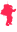Documentation

## Query Handler

The query handler controls how Nitrogen's `wf:q` and its siblings (`wf:qs`, `wf:mq`, `wf:mqs`) retrieves values from POST, GET, or other methods.

### Behavior Functions

##### `init(Config, State)`

Initialize the handler

• /Return Value/ - `{ok, NewState}`
##### `finish(Config, State)`

Clean up the handler

• /Return Value/ - `{ok, NewState}`
##### `get_value(Key, Config, State)`

This function should define how a querystring (GET) or form (POST) value is retrieved based on the provided `Key`. Note: this assumes a single value will be found for the provided key.

• `Key` - The key to find

• /Return Value/ - The value found for the provided `Key`.

• /Note:/ This will throw an error if more than one value is found for the `Key`

##### `get_values(Key, Config, State)`

This function should define how a querystring (GET) or form (POST) value is retrieved based on the provided `Key`. Note: this works

• `Key` - The key to find

• /Return Value/ - Description of the return

##### `get_params(Config, State)`
• /Return Value/ - This function returns an Erlang proplist (a list of key-value pairs, ie `[{Key,Value},...]`) of all the request variables and their values.

### Example

Here is the complete text of the default_query_handler:

``````
-module (default_query_handler).
-behaviour (query_handler).
-include_lib ("wf.hrl").
-export ([
init/2,
finish/2,
get_value/3,
get_values/3,
get_params/2
]).

init(_Config, _State) ->
% Get query params and post params
% from the request bridge...
RequestBridge = wf_context:request_bridge(),
QueryParams = RequestBridge:query_params(),
PostParams = RequestBridge:post_params(),

Params = QueryParams ++ PostParams,

% Pre-normalize the parameters.
Params1 = [{normalize_path(Path), Value} || {Path, Value} <- Params, Path /= undefined, Path /= []],
{ok, Params1}.

finish(_Config, _State) ->
% Clear out the state.
{ok, []}.

%% Given a path, return the value that matches the path.
get_value(Path, Config, State) ->
case get_values(Path, Config, State) of
[] -> undefined;
[One] -> One;
_Many -> throw({?MODULE, too_many_matches, Path})
end.

get_values(Path, _Config, State) ->
Params = State,
Path1 = normalize_path(Path),
refine_params(Path1, Params).

get_params(_Config, State) ->
Params = State,
F = fun({KeyPartsReversed, Value}) ->
KeyParts = lists:reverse(KeyPartsReversed),
Key = string:join(KeyParts, "."),
{ Key, Value }
end,
lists:map(F, Params).

%% Next, narrow down the parameters by keeping only the parameters
%% that contain the next element found in path, while shrinking the
%% parameter paths at the same time.
%% For example, if:
%%      Path   = [a, b, c]
%%      Params = [{[x, a, y, b, c], _}]
%% Then after the first round of refine_params/2 we would have:
%%   Path   = [b, c]
%%   Params = [y, b, c]
refine_params([], Params) ->
[V || {_, V} <- Params];
refine_params([H|T], Params) ->
F = fun({Path, Value}, Acc) ->
case split_on(H, Path) of
{ok, RemainingPath} -> [{RemainingPath, Value}|Acc];
false -> Acc
end
end,
Params1 = lists:foldl(F, [], Params),
refine_params(T, lists:reverse(Params1)).

split_on(_,  []) -> false;
split_on(El, [El|T]) -> {ok, T};
split_on(El, [_|T]) -> split_on(El, T).

normalize_path(Path) when is_atom(Path) ->
normalize_path(atom_to_list(Path));

normalize_path(Path) when ?IS_STRING(Path) ->
Tokens = string:tokens(Path, "."),
Tokens1 = [strip_wfid(X) || X <- Tokens],
lists:reverse(Tokens1).

strip_wfid(Path) ->
case Path of
"wfid_" ++ S -> S;
S -> S
end.

``````

Copyright © 2008-2021 Nitrogen Web Framework.Released under the MIT License.